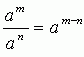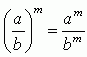Name: ___________________Date:___________________

 Email us to get an instant 20% discount on highly effective K-12 Math & English kwizNET Programs!

### High School Mathematics - 21.16 Properties of Exponents

 Laws of exponents: am x an = am+n (am)n = amn (ab)m = am x bma0 = 1 a1 = a a-n = 1/an Direction: Solve the following problems. Also write the laws of exponents on a piece of paper.
 Q 1: Simplify, [(x)7]2 = _____?x14x7 Q 2: Write the coefficient of x in (8/5)x?8/58 Q 3: Find the value of a7 when a=1?71 Q 4: Simplify, 4x2.2x3 = _____?(8x)58x5 Q 5: Write a.a.a in exponent form?a33a Q 6: Find the value of 3(2x-1), when x=9?5145 Q 7: Simplify, x2.9x3 = _____?9x5(9x)5 Q 8: Simplify, [(2x)2]2 = _____?16x416x2 Question 9: This question is available to subscribers only! Question 10: This question is available to subscribers only!